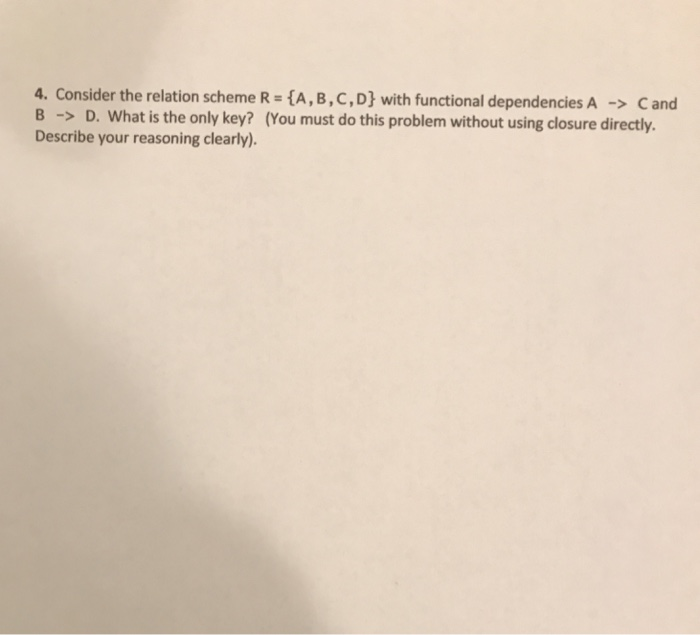# Question & Answer: Consider the relation scheme R = {A, B, C, D} with functional dependencies A rightarrow C and B rightarrow D. What i…..Consider the relation scheme R = {A, B, C, D} with functional dependencies A rightarrow C and B rightarrow D. What is the only key? (You must do this problem without using closure directly. Describe your reasoning clearly).

R = { A,B,C,D}

Don't use plagiarized sources. Get Your Custom Essay on
Question & Answer: Consider the relation scheme R = {A, B, C, D} with functional dependencies A rightarrow C and B rightarrow D. What i…..
GET AN ESSAY WRITTEN FOR YOU FROM AS LOW AS \$13/PAGE

A -> C

B -> D

A determines C and B determines D, so only key should be composite key(A,B) in the relation R

There will be partial dependencies ie C is partially dependent on (A,B) and D is also partially dependent on (A,B) . The partial dependencies can be removed by normalization .